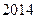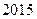Chapter 3, Problem 5SCQ### Principles of Economics 2e

2nd Edition
Steven A. Greenlaw; David Shapiro
ISBN: 9781947172364

#### Solutions

Chapter
Section### Principles of Economics 2e

2nd Edition
Steven A. Greenlaw; David Shapiro
ISBN: 9781947172364
Textbook Problem

# Let’s think about the market for air travel. From Augustto Januarythe price of jet fuel increased roughlyUsing the four-step analysis, how do you think this fuel price increase affected the equilibrium price and quantity of air navel?

To determine

Suppose the price of jet fuel increased roughly 47%. Use the four step analysis to describe the impact of increase in oil prices on equilibrium quantity and price level.

Explanation

If the price of jet fuel increased roughly by 47% then, The four step analysis is given below:

(a) The demand and supply diagram is given below for air travel.

The demand and supply curve for air travel is shown in the above diagram. The equilibrium price and quantity is ‘P’ and ‘Q’ respectively.

(b) The jet fuel is used as an input in air travel and it affects the supply of air travel

(c) An increase in the price of jet fuel will affect the supply of air travel adversely...

### Still sussing out bartleby?

Check out a sample textbook solution.

See a sample solution

#### The Solution to Your Study Problems

Bartleby provides explanations to thousands of textbook problems written by our experts, many with advanced degrees!

Get Started

#### Find more solutions based on key concepts